# Intermediate Microeconomics Exam 3 Flashcards

Set Details Share
created 7 years ago by Myles_Shealey
3,026 views
updated 7 years ago by Myles_Shealey
Page to share:
Embed this setcancel
COPY
code changes based on your size selection
Size:
X

1

The production function describes:

a. the relationship between firm inputs and firm output

b. the relationship between firm inputs and firm profits

c. the relationship between firm production and production costs

d. the relationship between firm production and firm profits

A

2

Which of the following are examples of production functions?I. Q=56K+18L

II. Q = 2K0.8L0.2

III. Q = KL

a. II and III

b. I, II, and III

c. II only

d. I only

B

3

Diminishing marginal product of labor means that:

a. total output falls as more labor is employed

b. the increments to output produced by successive units of labor (holding other inputs constant) declines

c. as more capital is employed, the marginal product of labor declines

d. the increments to output produced by successive units of labor (holding other inputs constant) becomes negative

B

4

A donut shop has a production function given by q = 50K1/3L1/2, where q is the number of donuts produced per hour, K is the number of donut fryers (which is fixed at eight in the short run), and L is the number of employed workers. How many donuts can be produced per hour with four workers in the short run?

a. 200

b. 167

c. 320

d. 84

A

5

The short-run production function for a firm is given by q = 40L2/3. What is the average product of labor?

a. APL = 16.67L

b. APL = 40/L1/3

c. APL = 40/L

d. APL = 1/40L

C

6

Brenda's Pastry employs three workers who produce 6 dozen pastries per hour. After Brenda hires a fourth worker, production increases to 7 dozen pastries per hour. Which of the following statements is TRUE?

a. Because the marginal product of the fourth worker is greater than the average product of three workers, the average product must fall.

b. Because the marginal product of the fourth worker is less than the average product of three workers, the average product must rise.

c. Because the marginal product of the fourth worker is greater than the average product of three workers, the average product must rise.

d. Because the marginal product of the fourth worker is less than the average product of three workers, the average product must fall

D

7

In the long run:

a. At least one input is variable

b. At least one input is fixed

c. All inputs are variable

d. All inputs are fixed

C

8

An isoquant depicts:

a. all combinations of inputs that are of equal cost to the firm

b. all combinations of inputs that produce the same output level

c. all combinations of inputs that minimize production costs for various output levels

d. none of the above

B

9

Suppose that a community health center finds that, regardless of the number of physicians or nurse practitioners employed, it can always replace one family practice physician by using two additional nurse practitioners without affecting the quantity (and quality) of patient office visits. This finding implies that:

a. physicians and nurse practitioners are perfect substitutes in production.

b. physicians and nurse practitioners are perfect complements in production.

c. diminishing returns will occur as the health center hires more nurse practitioners.

d. the marginal product of physicians will increase by two with every nurse practitioner hired.

A

10

True or False? Diminishing MRTS (of L for K) implies that the MRTS is higher when the capital-labor ratio is higher.

a. T

b. F

A

11

The production function given by q = 10K2 + L2 has ______ returns to scale.

a. constant

b. instant

c. increasing

d. decreasing

C

12

A firm finds that when it triples all of its inputs, output doubles. The firm’s production function exhibits:

a. increasing returns to scale

b. constant returns to scale

c. decreasing returns to scale

d. diminishing marginal product

C

13

For every one machine Firm Alpha employs, it must employ 2 (and only 2) workers. There is no possibility for substitutions among inputs. Which of the following statements is/are correct?

I. Firm Alpha has a fixed-proportions production function

II. Firm Alpha’s isoquants are L-shaped

III. Firm Alpha’s output will be the same if it employs 4 machines and 8 workers or it employs 4 machines and 20 workers

IV. Firm Alpha’s output will be the same if it employs 3 machines and 8 workers or it employs 4 machines and 8 workers

a. I only

b. I and II only

c. I, II and III only

d. I, II, III and IV

C

14

Costs that change as production changes are

a. Fixed costs

b. Sunk costs

c. Variable costs

d. Standard costs

C

15

Economic profit is

a. The total revenue minus explicit costs

b. The total revenue minus implicit costs

c. The total revenue plus implicit costs

d. The total revenue minus both implicit and explicit costs

D

16

Accounting profit is

a. Total revenue minus implicit costs

b. Total revenue minus both implicit and explicit costs

c. Total revenue minus explicit costs

d. Total revenue plus explicit costs

C

17

Marginal cost is computed by

a. Dividing total cost by output

b. Dividing total variable cost by output

c. Dividing the changes in total cost by output

d. Dividing the change in total cost by the change in output

D

18

The marginal cost curve will

a. Intersect the average variable cost curve at its highest point

b. Intersect the average fixed cost curve at its lowest point

c. Intersect the average total cost curve at its highest point

d. Intersect the average total cost curve at its lowest point

D

19

The relationship between marginal cost and marginal product is

a. If marginal product is falling marginal cost is rising

b. If marginal product is falling, marginal cost is falling

c. If marginal product is less than marginal cost always

d. Marginal product equals marginal cost always

A

20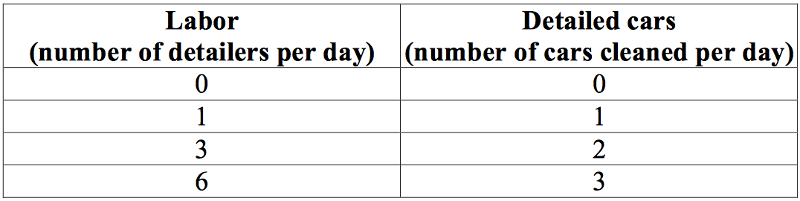(Table 7.2) Carl's Detailing is a small business that travels to people's homes to meticulously clean their high-end sports cars. Carl hires detailers (labor) at a wage of \$80 per day per worker. A van that is used to carry cleaning supplies to client homes has a fixed cost of \$20 per day. The table shows the relationship between the number of cars cleaned and the number of detailers. The marginal cost of cleaning the first car is ______, and the marginal cost of cleaning the third car is ______.

a. \$80; \$480

b. \$80; \$240

c. \$100; \$260

d. \$100; \$300

B

21

When graphing total fixed cost, the graph would be

a. A straight vertical line

b. A straight horizontal line

c. A straight line with a positive slope

d. A straight line with a negative slope

B

22

The difference between total cost and total variable cost is

a. Marginal cost

b. Total fixed cost

c. Average fixed cost

d. Average variable cost

B

23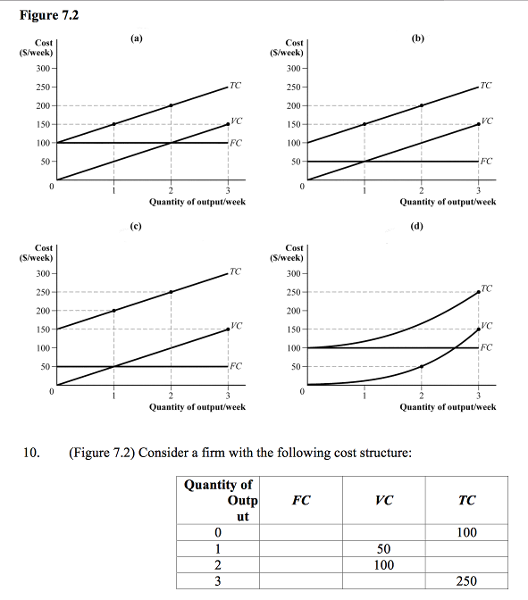Which of the preceding represents the cost curves of the firm?

a. panel (a)

b. panel (b)

c. panel (c)

d. panel (d)

A

24

A firm is producing 50 units of output at a total cost of \$1,000, with a per-unit variable cost of \$8. What is the firm's average fixed cost?

a. \$4

b. \$12

c. \$20

d. \$28

B

25

(Table 7.1) Which of the following is correct at 4 units of output?

a. AFC = 70; AVC = 21; ATC = 91

b. AFC = 17.50; AVC = 52.50; ATC = 70

c. AFC = 0.06; AVC = 0.02; ATC = 0.08

d. AFC = 280; AVC = 840; ATC = 1,320

B

26

Suppose a firm's total cost is given by TC = 100 + 4Q + 2Q2. Which of the following statements is TRUE?

I. AVC = 4Q + 2Q2

II. AFC = 100/Q

III. ATC = 2Q + 4 + 100/Q

IV. FC = 100 + 4Q

a. I and II

b. II and III

c. III only

d. I and IV

B

27

Suppose a firm's total costs are given by TC = 150 + 0.50Q +1.5Q2. What is the firm's average variable cost of producing 10 units of output?

a. \$5.55

b. \$15.50

c. \$15

c. \$17

B

28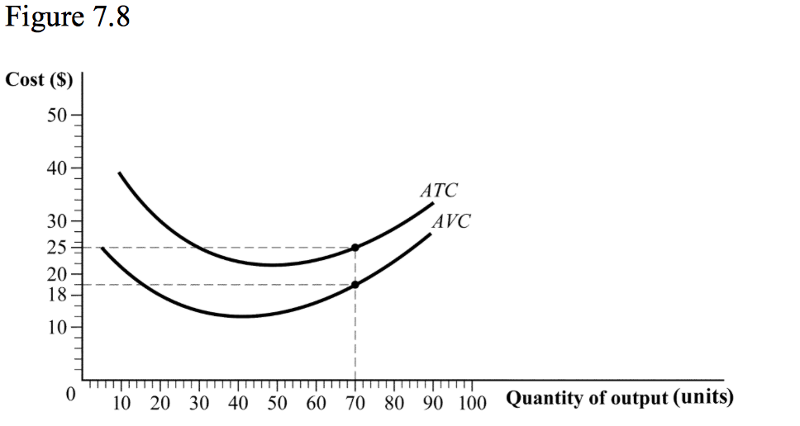(Figure 7.8) The average fixed cost at 70 units of output is ______, and the firm's fixed cost of production is ______.

a. \$43; \$3,010

b. \$11; \$560

c. \$0.10; \$7

d. \$7; \$490

D

29

A firm can produce widgets as follows: q = 6√K*L. In the short run, capital is fixed at 4 units, the wage rate is \$10 and the rental rate on capital is \$10. In the short run, to produce 60 units of output, the firm will use ___ workers and total costs will be ___.

a. 16; \$200

b. 25; \$250

c. 25; \$290

d. 100; \$290

C

30

When average cost is falling, marginal cost is ____________ and when average cost is rising, marginal cost is _________________.

a. falling, above average cost

b. above average cost, below average cost

c. below average cost, above average cost

d. both a and c

C

31

Which of the following explains why short run marginal costs will always eventually rise?

a. decreasing returns to scale

b. increasing returns to scale

c. diminishing marginal product

d. diminishing marginal rate of technical substitution

C

32

Generally speaking, the short-run average cost curve is __________________ with short-run marginal cost _____________.

a. horizontal, equal to short-run average cost

b. always increasing, above short-run average cost

c. U-shaped, greater than short-run average cost

d. U-shaped, intersecting short-run average cost from below at its minimum

D

33

An increase in the wage rate will shift the firm’s cost curves ______________, the extent of the shift being larger the ____________ important labor is in the firm’s production and the ____________ substitutable capital is for labor.

a. upward, more, more

b. upward, more, less

c. upward, less, more

d. downward, more, less

B

34

The difference between the short run and the long run is

a. In the short run all costs are variable

b. In the long run all costs are fixed

c. In the long run at least one cost is fixed

d. In the short run at least one cost is fixed

D

35

Suppose the wage rate is \$10 and the rental rate on capital is \$20. Consider the isocost line associated with a total cost of \$200. The intercept of this line on the Y-axis (capital axis) is ______________ and its slope is _____:

a. 20, −1⁄2

b. 10, −2

c. 20, −2

d. 10, −1⁄2

D

36

Given the usual-shaped isoquants, at the cost- minimizing input combination for producing some output level q1,

a. the isocost line is tangent to the q1 isoquant

b. the MRTS (of L for K) is equal to w/v

c. MPL/ MPK is equal to w/v

d. all of the above

D

37

In the long run, a firm chooses its levels of capital and labor to minimize costs. If the rental rate for capital is \$20 per hour and the wage rate for labor is \$10 per hour, then to minimize costs, Alpha should choose capital and labor such that the marginal rate of technical substitution (MRTS of L for K) is equal to:

a. 1/2

b. 2

c. 10

d. none of the above

A

38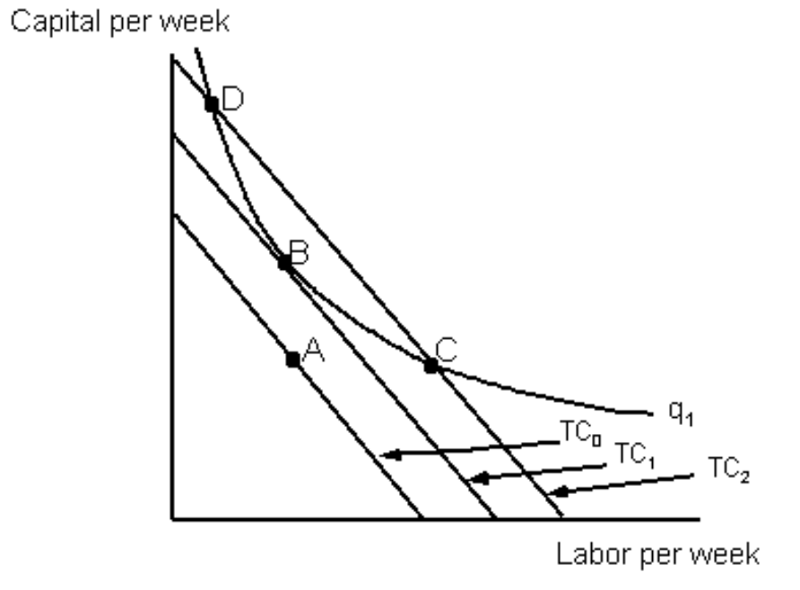The figure below represents a firm wishing to produce q1 units of output. Which of the following statements is/are correct?

I. Input combination A costs less than input combination B, but A is insufficient for producing an output level of q1

II. Both input combinations B and C will produce an output level of q1, but C has a higher total cost than B

III. The firm’s optimal input combination for producing q1 is B

IV. Both input combinations C and D produce an output level of q1, but D has a higher total cost than C

a. I and II only

b. I and III only

c. I, II and III only

d. I, II, III and IV

C

39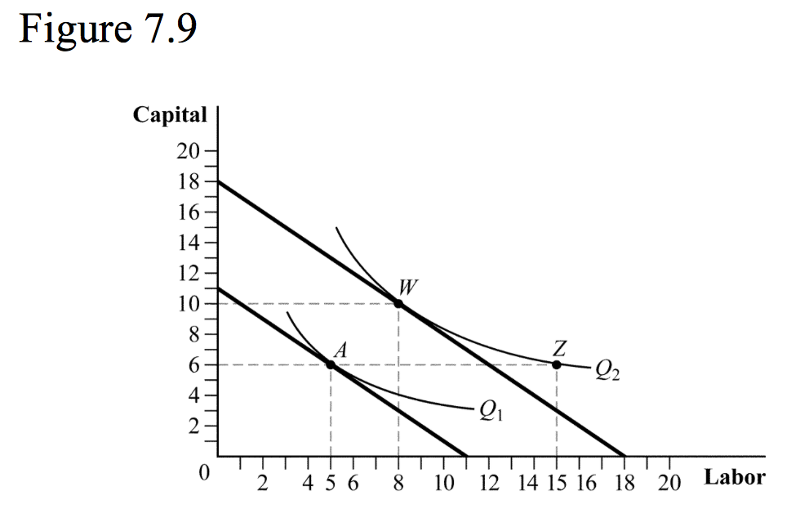(Figure 7.9) Suppose the firm is currently producing at point A. If the firm wants to produce more output, it will:

a. not be able to because capital is fixed.

b. move to point W in the short run and point Z in the long run.

c. move to a point between W and Z in the short run.

d. move to point Z in the short run and point W in the long run.

D

40

In the long run, a firm is producing some output level q1 using a combination of capital and labor for which the MRTS (of L for K) at this input combination is 1. Suppose further that the wage rate is \$10 and the rental rate on capital is \$20. Which of the following statements is correct?

a. The firm can reduce its costs of producing q1 by using more labor and less capital

b. The firm can reduce its costs of producing q1 by using more capital and less labor

c. The firm is currently minimizing the costs of producing q1

d. Whether or not the firm is minimizing the costs of producing q1 is indeterminate

A

41

To construct the long run average cost curve

a. Short run average variable cost curves are averaged

b. Short run fixed cost curves are averaged

c. Marginal costs curves are averaged

d. Short run average total cost curves are used

D

42

(Be careful) A firm is producing 10,000 units of output at a total cost of \$5,000. If the firm increases output by 5,000 units and its total costs rise by \$2,000, the firm is experiencing:

a. economies of scale.

b. economies of scope.

c. constant returns to scale.

d. diseconomies of scale.

A